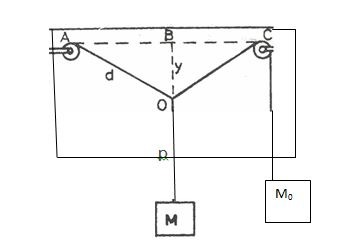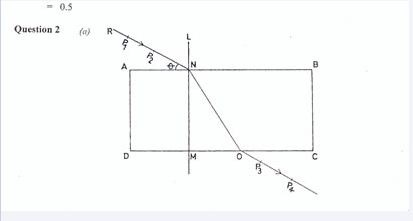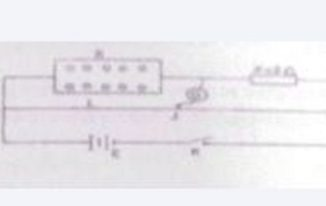# Get NECO GCE 2024 Physics Practical Questions and Answers

Are you Searching for NECO 2024/2025 GCE Physics questions and Answers? If yes, you're on the right place.

The NECO GCE physics practical 2024 questions and answers are now available. The National Examination Council (NECO) Senior School Certificate Exam (SSCE) Physics Practical paper will be written on Monday 29th November 2024.

The NECO Physics Practical exam will commence from 2:00 pm to 4:45 pm. The Neco physics practical is usually divided into 3 questions (comprising of Mechanics, Waves, and Electricity) with a few other general knowledge calculations added.

I will also show a sample of how the exam will look including possible questions and answers which will enable you to practice and get ready to score high.

## Materials and apparatus for the exam

The provided materials and apparatus for the practical physics exam are as follows;

1. Two Retort stands, Drawing board, Fixed Pulley, Drawing papers, Four Thumb pins, Masses (30,20,10, and 5g), Mass =30g covered and labeled W, Mass hanger, and Twine.
2. Rectangular glass prism, Four optical pins, Drawing board, Drawing papers, and Four Thumb pins.
3. 2Ω standard resistor covered and labeled X, Resistance box, Meter bridge, Galvanometer, Jockey, 2V accumulator or two × 1.5V laclanche cells, Key, and connecting wires.

## NECO GCE Physics Practical Questions 2021

QUESTION 1:

(a) DiagramUsing the above diagram as a guide, carry out the following instructions.
i. Attach the mass Mo to one end of the thread provided. Fix the other end of the thread to pulley a on the force board. Pass the thread through pulley C.
ii. Hang the force board vertically. Fix a drawing paper on the board behind the thread.
iii. Draw a line on the paper along the direction of AC. Locate and mark the mid point B of line AC.

(iv)Draw a normal to AC at B.

v. By means ofa loop of thread, suspend mass M = 70 g from AC. Adjust the position of the loop so that the line of action of the weight of M lies along BP, the normal to A C.
vi. Ensure that M and Mo hand off the force board. Trace the paths AO, OC and OP of the thread.

vii. Measure and record y = BO. Also measure and record d = AO.

viii. Evaluate 1 = y /d
ix. Repeat the procedure for other values of M = 100, 120, 150 and 170g.
xi. Plot a graph with I on the vertical axis and M on the horizontal axis.
xii. Determine the slope, s, of the graph.
xiii. State two precautions taken to ensure accurate results.

(b) i. State parallelogram law of vector addition.
ii. State the conditions necessary for a body to be in equilibrium when acted upon by a number
of parallel coplanar forces.

QUESTION 2:

(a) DiagramUse the diagram above as a guide to carry out the following experiment.

i. Trace the outline ABCD of the rectangular glass prism on the drawing paper provided.
ii. Remove the prism. Select a point N on AB such that AN is about one quarter of AB.
iii. Draw the normal LNM.  Also draw a line RN to make an angle θ = 75o with AB at N
iv. Fix two pins at P1 and P2 on line RN. Replace the prism on its outline.

v. Fix two other pins at P3 and P4 such that they appear to be in a straight line with the images of the pins at P1 and P2 when viewed through the prism from side DC.
vi. Remove the prism and the pins at P3 and P4 Draw a line to join P3 and P4.
vii. Produce line P4P3 to meet the line DC at O. Draw a line to join NO.
viii. Measure and record the values of MO and NO.
ix. Evaluate ф =  and  cos θ.

x. Repeat the procedure for four other values of θ – 65o, 55o, 45o and 35o. In each case, evaluate ф and cos θ
xii. Plot a graph with cos θ on the vertical axis and ф on the horizontal axis.
xiii. Determine the slope, s, of the graph.
xiv. Sate two precautions taken to ensure accurate results.

(b) i. State Snell’s law of refraction.
ii. Calculate the critical angle for the glass prism used in the experiment above if its refractive index is 1.5.

QUESTION 3:(a) You are provided with a meter bridge, standard resistor X galvanometer. jockey, cells, a key resistance box and some connecting wires.

i. Connect the circuit as shown above.
ii. With R set to 10 Q obtain a balance point J on VB
iii. Measure and record length AJ =L
iv. Evaluate L-1 and R-1
v. Repeat the procedure with values of R = 20 Ω, 30 Ω,  40 Ω, 50 Ω and 6O Ω
vi. In each case, measure and record L and evaluate L-1 and R-1
viii. Plot a graph with L-1 on the vertical axis and R-1 on the horizontal axis
ix. Determine the slope, s, of the graph and the intercept, i. on the vertical axis
x. Evaluate the quantities.
(α) Q, = 100s,
(β) Q2  = i-1
xi. State two precautions are taken to ensure accurate results.

(b) i. State Ohm’s law.
ii. Calculate the resistivity of a wire of length 1 0m* diameter 7 0 x 10“* m and electrical resistance of 1.00.

Related Posts:

NECO SSCE Practical Questions and answers

NECO SSCE Recent Questions and Answers

Please Select Embedded Mode To Show The Comment System.*